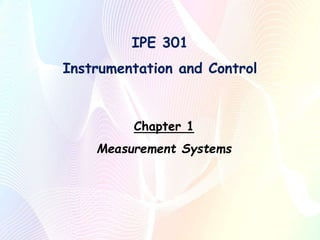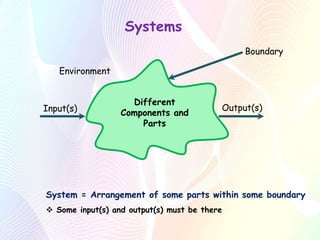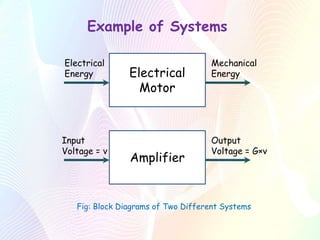Successfully reported this slideshow.

# Ch 01 (Measurement Systems).pptx×

# Ch 01 (Measurement Systems).pptx

An introductory slide on the topics of measurement and control. This elementary topics act as the gateway to advanced concepts of automation, instrumentation and programming production lines.

An introductory slide on the topics of measurement and control. This elementary topics act as the gateway to advanced concepts of automation, instrumentation and programming production lines.

### Ch 01 (Measurement Systems).pptx

1. 1. IPE 301 Instrumentation and Control Chapter 1 Measurement Systems
2. 2. Systems Different Components and Parts Boundary Input(s) Output(s) System = Arrangement of some parts within some boundary  Some input(s) and output(s) must be there Environment
3. 3. Example of Systems Electrical Motor Electrical Energy Mechanical Energy Amplifier Input Voltage = v Output Voltage = G×v Fig: Block Diagrams of Two Different Systems
4. 4. Interconnected Systems A B Input to A Final Output Output from A is input to B  Linked systems  Arrows generally represent physical connection  Arrows might represent flow of information instead of physical connection
5. 5. Case Study of Interconnected Systems: Processing Sequence for Frutika Fruit Drink
6. 6. Measurement System Measurement System Input: True value of a variable Output: Measured value of the variable  Give the user a numerical value corresponding to the variable being measured  The numerical value may not actually be the true value of the variable.
7. 7. Example of Measurement System Anemometer Input: Wind (speed) Output: Value for wind speed
8. 8. The Constituent Elements of an Instrumentation System A C B Temperature Signal Resistance Change Current Change Movement of pointer across a scale Fig: System Elements of a Resistance Thermometer
9. 9. The Constituent Elements of an Instrumentation System 1.Sensor  Detects events or changes in its environment  Provide a corresponding output  The output depends in some way on the value of the input variable  This output is used by the rest of the measurement system to give a value to it Example: Thermocouple
10. 10. 2.Signal Processor  Takes the output from the sensor  Converts the output into a form which is suitable for display Example: Amplifier The Constituent Elements of an Instrumentation System
11. 11. 3.Data Presentation  Presents the measured value in a form which enables an observer to recognize it Example: Display The Constituent Elements of an Instrumentation System
12. 12. Transducers  Converts a change in some physical variable into a related change in some other physical variable  It is generally used for an element that converts a change in some physical variable into an electrical signal change Example: Galvanometer, Electrical Motor Are sensors TRANSDUCERS? The Constituent Elements of an Instrumentation System
13. 13. Performance Terms Accuracy:  Maximum overall error to be expected from a measurement system  Accuracy is expressed as the inaccuracy and appears in several forms. (1) Measured variable (2) Percentage of the instrument full-scale (FS) (3) Percentage of instrument range or span (4) Percentage of the actual reading *FS=maximum value of the span or Reference
14. 14. Example: A temperature sensor has a span of 10°-300°C. A measurement results in a value of 100°C for the temperature. Specify the error if the accuracy is (a) ± 4°C of measured value, (b) ± 0.5% FS, (c) ± 0.75% of span, and (d) ± 0.8% of reading. Solution: (a) Error = ± 4°C (b) Error = ± (0.5% of 300°C) = ± 1.5°C (c) Error = ± {0.75% of (300 ˗ 10)°C} = ± 2.175°C (d) Error = ± (0.8% of 100°C) = ± 0.8°C  Magnitude of Error = |Measured Value – True Value|
15. 15. Errors in Specifications of Instrumentation Systems Hysteresis Error: Value Measured Instrument Reading Increasing Decreasing Hysteresis Error
16. 16. Errors in Instrumentation Systems Non-linearity Error: Input Output Assumed Linearity Actual Relationship Non-linearity Error
17. 17. Errors in Instrumentation Systems Insertion Error: Most of the time the act of attempting to make the measurement modified the value of the variable being measured. This effect is called loading and the consequence as an insertion error. Example:  Cold thermometer in a hot liquid  Ammeter inserted into a circuit  Voltmeter connected across a resistor
18. 18. Errors in Instrumentation Systems Problem: Two voltmeters are available, one with a resistance of 1 kΩ and the other 1 MΩ. Which instrument should be selected if the indicated value is to be closest to the voltage value that existed across a 2 kΩ resistor before the voltmeter was connected across it?
19. 19. Performance Terms Dead Band or Dead Space: Output Input Dead Band  A range of input values for which there is no output
20. 20. Performance Terms Precision:  Describes the degree of freedom of a measurement system from random errors  High precision measurement instrument will give only a small spread of readings if repeated readings are taken of the same quantity.  A low precision measurement system will give a large spread of readings. Example: Readings from instrument 1: 17.5, 18.3, 19, 18.5, 20.2 Readings from instrument 2: 18.2, 18.3, 18.5, 18.5, 18.2
21. 21. Performance Terms Repeatability:  The ability of a measurement system to give the same value for repeated measurements of the same value of a variable.  Cause of non-repeatability: Random fluctuations in the environment, e.g. changes in temperature, humidity etc. Reproducibility:  Ability of the system to give the same output when it and/or elements of the system are disconnected from the input and then reinstalled.  The resulting error (from both repeatability and reproducibility) is usually expressed as a percentage of the full range output.
22. 22. Performance Terms Difference between Accuracy and Precision:
23. 23. Performance Terms Sensitivity:  Indicates how much the output of an instrument system or system element changes when the quantity being measured changes by a given amount.  Example: A thermocouple having a sensitivity of 20 µV/°C gives an output of 20 µV for each 1°C change in temperature.  Also used to indicate the sensitivity to inputs other than that being measured.  A pressure measurement sensor might be quoted as having a temperature sensitivity of ±0.1% of the reading per °C change in temperature.
24. 24. Performance Terms A pressure measurement system is stated as having the following characteristics. Explain the significance of the terms: Range: 0 to 125 kPa and 0 to 2500 kPa Accuracy: ±1% of the displayed reading Temperature sensitivity: ±0.1% of the reading per °C
25. 25. Reliability Reliability of a measurement system:  The probability that the system will operate to an agreed level of performance, for a specified period, subject to specified environmental conditions.  The reliability is likely to change with time.  A high reliability system will have a low failure rate.  Failure rate is the number of times during some period of time that the system fails to meet the required level of performance.
26. 26. Requirements Requirements of a measurement system:  The main requirement is fitness for purpose.  In order to deliver the required accuracy, the measurement system must have been calibrated to give that accuracy.  Calibration is the process of comparing the output of a measurement system against output from a standard measurement system of known accuracy.  Standard measurement systems are kept specially for calibration duties or some means of defining standard values.